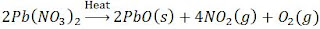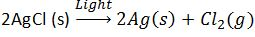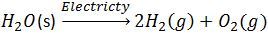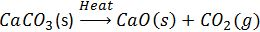## Extra Questions for Class 10th Science: Ch 1 Chemical Reactions and Equations| Important Question Answer Included

Very Short Answer Questions (VSAQs): 1 Mark

Q1. What happens when magnesium ribbon burns in air?

When magnesium ribbon burns in air, it combines with the oxygen to form magnesium oxide.
2Mg(s) + O2 (g) → 2MgO(s)

Q2. On what chemical law, balancing of chemical equation is based?

Balancing of a chemical equation is based on the law of conservation of mass.

Q3. Name the gas evolved when zinc reacts with dil. HCl.

Hydrogen gas is evolved.

Q4. Name and state the law which is kept in mind while we balance a chemical equation.

Law of conservation of mass. Mass can neither be created nor be destroyed during a chemical reaction.

Q5. Write the chemical equation for reactions that takes place when lead nitrate and potassium iodide solutions are mixed.

Pb(NO3)2 + 2KI → 2KNO3 + PbI2

Q6. Why is photosynthesis considered an endothermic reaction?

The photosynthesis is considered an endothermic reaction because heat is absorbed in this process.

Q7. What is percipitate?.

Percipitate is insoluble metal compound formed after reaction.

Short Answer Questions-I (SAQs-I): 2 Marks

Q1. Why does the colour of copper sulphate solution change when an iron nail is dipped in it? Write chemical equation for the reaction involved.

Iron is more reactive than copper so it displaces copper from copper sulphate solution and forms iron sulphate, hence the colour of solution changes.
Equation: Fe + CuSO4 (aq) → FeSO4 (aq) + CuO

Q2. State reason for the following:
(i) Potato chips manufactures fill the packet of chips with nitrogen gas.
(ii) Iron articles are shining when new, but get coated with a reddish brown powder, when left for some time.

(i) Potato chips manufactures fill the packet of chips with nitrogen gas to prevent rancidity.
(ii) Rusting and corrosion.

Q3. A student prepares aqueous solutions of the following salts: Copper sulphate : ferrous sulphate, Sodium sulphate, barium chloride. Write the colour of each solution thus formed.

Copper sulphate — Green
Ferrous sulphate — Pale green
Sodium sulphate — Milky white
Barium chloride — White

Q4. Barium chloride reacts with aluminium sulphate to give, aluminium chloride and barium sulphate.
(i) State the two types in which the above reaction can be classified.
(ii) Translate the above statement into a chemical equation.

(i) Double displacement reaction and Precipitation reaction.
(ii) 3BaCl2 + Al2(SO4)3 → 2AlCl3 + 3BaSO4(↓)

Short Answer Questions-II (SAQs-II): 3 Marks

Q1. Write one equation each for decomposition reactions where energy is supplied in the form of heat, light and electricity.

(i) Decomposition by heat of lead nitrate.(ii) Decomposition by light of Silver Chloride(iii) Decomposition by electricity of WaterQ2. State the kind of chemical reactions in the following examples in terms of loss and gain of energy:
(i) Digestion of food in stomach
(ii) Combustion of coal in air
(iii) Heating of limestone.

Digestion of food in Stomach
C6H12O6 + 6O2 → 6CO2 + 6H2O + Energy
Energy is released in the process. It is exothermic reaction.
Combustion of Coal
Coal is Carbon. It is a combustion reaction.
C+O2→CO2
Energy is released in the process hence it is known as exothermic reaction.
Heating of Limestone.
Limestone is calcium carbonate. Ca2CO3Energy is absorbed in the process. So, the reaction is endothermic reaction.

Q3A solution of a substance 'X' is used for testing carbon dioxide. Write the equation of the reaction of X with carbon dioxide. (b) How is X obtained? Write chemical equation.

(a) Substance X is lime water which is aqueous solution of Calcium Hydroxide.
Ca(OH)2 (aq)+CO2 (g)→CaCO3 (s)+H2O(l)
When limewater reacts with Carbon di oxide, it turns milky, that is Calcium Hydroxide.

(b) Calcium oxide reacts vigorously with water to produced slaked time (calcium hydroxide) releasing a large amount of heat.Q4. Write the steps for balancing the chemical equation for the formation of ammonia by the combination of nitrogen and hydrogen.

First, we write the skeletal chemical equation for the process.
N2+H2→NH3
Examine the number of atoms of different elements present in the unbalanced equation.
In the above reaction both the sides Nitrogen and Oxygen both are unbalanced.
To balance hydrogen, H2 is multiplied by 3 LHS. It makes 6H-atoms on the left hand side.
Now, to balance hydrogen, in RHS, 2 is multiplied to NH3.
Nitrogen is balanced automatically.
N2 (g)+3H2 (g)→2NH3 (g)

Long Answer Questions (LAQs): 5 Marks

Q1. (a) Explain the term "rancidity." Name the type of chemical reaction responsible for causing rancidity and define it. (b) Write three methods for preventing rancidity of food.

We have seen food getting contaminated and having an unpleasant smell if it is left open in summer.  In a hot weather, Oil present in food get oxidized and become Rancid. Such food is not recommended for consumption.
The food is getting oxidized so the reaction is oxidation reaction.
Methods for preventing food from getting oxidized are:-
(a) Food is kept in cool place like refrigerator.
(b) Packaging should be air tight
(c) Antioxidants should be added to food.

Q2. (a) List any three observations that determine that a chemical reaction has taken place. Also list three informations that cannot be obtained about a chemical reaction, merely by its chemical equation.
(b) Balance the following chemical equations.
(i) Fe + H2O → Fe3O4 + H2
(ii) CO2 + H2O → C6H12O6+O2